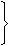# Topic: Basic Numeracy (Test 2)

Topic: Basic Numeracy
Q.1
What was the day of the week on 28th May, 2006?
A. Thursday
B. Friday
C. Saturday
D. Sunday
Explaination / Solution:

28 May, 2006 = (2005 years + Period from 1.1.2006 to 28.5.2006)

Odd days in 1600 years = 0

Odd days in 400 years = 0

5 years = (4 ordinary years + 1 leap year) = (4 x 1 + 1 x 2)  6 odd days

Jan.  Feb.   March    April    May
(31 +  28  +  31   +   30   +   28 ) = 148 days
148 days = (21 weeks + 1 day)  1 odd day.

Total number of odd days = (0 + 0 + 6 + 1) = 7  0 odd day.

Given day is Sunday.

Workspace
Report
Q.2
In the first 10 overs of a cricket game, the run rate was only 3.2. What should be the run rate in the remaining 40 overs to reach the target of 282 runs?
A. 6.25
B. 6.5
C. 6.75
D. 7
Explaination / Solution:

 Required run rate =282 - (3.2 x 10)= 250 = 6.25 40 40

Workspace
Report
Q.3
A grocer has a sale of Rs. 6435, Rs. 6927, Rs. 6855, Rs. 7230 and Rs. 6562 for 5 consecutive months. How much sale must he have in the sixth month so that he gets an average sale of Rs. 6500?
A. Rs. 4991
B. Rs. 5991
C. Rs. 6001
D. Rs. 6991
Explaination / Solution:

Total sale for 5 months = Rs. (6435 + 6927 + 6855 + 7230 + 6562) = Rs. 34009.Required sale = Rs. [ (6500 x 6) - 34009 ]

= Rs. (39000 - 34009)

= Rs. 4991.

Workspace
Report
Q.4
In a shower, 5 cm of rain falls. The volume of water that falls on 1.5 hectares of ground is:
A. 75 cu. m
B. 750 cu. m
C. 7500 cu. m
D. 75000 cu. m
Explaination / Solution:

1 hectare = 10,000 m2

So, Area = (1.5 x 10000) m2 = 15000 m2.

 Depth = 5 m = 1 m. 100 20Volume = (Area x Depth) =15000 x 1m3 = 750 m3. 20

Workspace
Report
Q.5
A hall is 15 m long and 12 m broad. If the sum of the areas of the floor and the ceiling is equal to the sum of the areas of four walls, the volume of the hall is:
A. 720
B. 900
C. 1200
D. 1800
Explaination / Solution:

2(15 + 12) x h = 2(15 x 12)h = 180 m = 20 m. 27 3Volume =15 x 12 x 20m3 = 1200 m3. 3

Workspace
Report
Q.6
Find the greatest number that will divide 43, 91 and 183 so as to leave the same remainder in each case.
A. 4
B. 7
C. 9
D. 13
Explaination / Solution:

Required number = H.C.F. of (91 - 43), (183 - 91) and (183 - 43)

= H.C.F. of 48, 92 and 140 = 4.

Workspace
Report
Q.7
The H.C.F. of two numbers is 23 and the other two factors of their L.C.M. are 13 and 14. The larger of the two numbers is:
A. 276
B. 299
C. 322
D. 345
Explaination / Solution:

Clearly, the numbers are (23 x 13) and (23 x 14).Larger number = (23 x 14) = 322.

Workspace
Report
Q.8
(17)3.5 x (17)? = 178
A. 2.29
B. 2.75
C. 4.25
D. 4.5
Explaination / Solution:

Let (17)3.5 x (17)x = 178.

Then, (17)3.5 + x = 178.3.5 + x = 8x = (8 - 3.5)x = 4.5

Workspace
Report
Q.9
Given that 100.48 = x, 100.70 = y and xz = y2, then the value of z is close to:
A. 1.45
B. 1.88
C. 2.9
D. 3.7
Explaination / Solution:

xz = y210(0.48z) = 10(2 x 0.70) = 101.400.48z = 1.40z = 140 = 35 = 2.9 (approx.) 48 12

Workspace
Report
Q.10
3 pumps, working 8 hours a day, can empty a tank in 2 days. How many hours a day must 4 pumps work to empty the tank in 1 day?
A. 9
B. 10
C. 11
D. 12
Explaination / Solution:

Let the required number of working hours per day be x.

More pumps, Less working hours per day (Indirect Proportion)

Less days, More working hours per day (Indirect Proportion)

 Pumps 4 : 3:: 8 : x Days 1 : 24 x 1 x x = 3 x 2 x 8x = (3 x 2 x 8) (4)x = 12.

Workspace
Report